<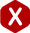# Coil Spring Dimensions

#### Definition: The measurements or sizing of different parts of a helical coil spring.

Your coil spring's dimensions are what make up your coil spring. From outer diameter to wire diameter, free length, total coils; even material type. All of these spring dimensions are used to calculate your spring’s rate, max travel, and working loads.

#### Coil Compression Spring Dimensions:

The coil compression spring dimensions to calculate the force and elasticity of your spring are the following:

• Outer Diameter
• Inner Diameter
• Wire Diameter
• Mean Diameter
• Free Length
• Total Coils
• Active Coils
• Material Type
• Spring Index
• Type of Ends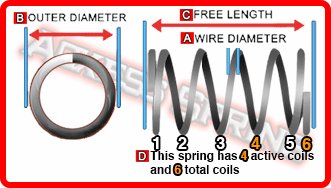On our coil compression spring calculator, Spring Creator, we will only ask you for outer diameter, wire diameter, free length, total coils, material type and end type because the other dimensions like rate and working loads can be calculated using these. If your spring will be placed inside a hole or over a shaft, be sure to input a value which is at least 0.015” larger or smaller depending on whether it is a hole or shaft. The reason for this is because there has to be a little room in between your spring’s coils and the walls of the hole or shaft in order to compress safely without friction against the walls.

#### Coil Extension Spring Dimensions

The coil extension spring dimensions needed to calculate its force and elasticity are the following:

• Outer Diameter
• Inner Diameter
• Wire Diameter
• Mean Diameter
• Length Inside Hooks
• Body Length
• Hook Length
• Active Coils
• Material Type
• Spring Index
• Type of Hooks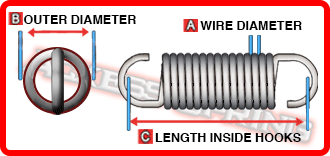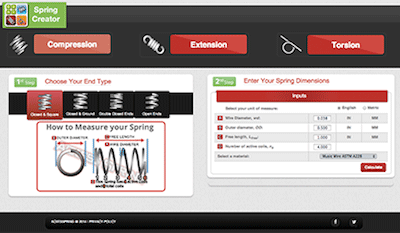In the case of a coil tension spring, Spring Creator will only ask that you input wire diameter, outer diameter, length inside hooks, material type and hook type (hook lengths if extended hooks are selected). Once these coil spring dimensions are input into the calculator and you have hit the calculate button, the other coil spring dimensions will automatically be calculated as well as your coil tension spring’s rate, maximum loads and travel, and initial tension. In order for your spring to be steady and not move around in your mechanism, you must make sure that your coil tension spring’s length inside hooks dimension is a bit shorter than the length in between the support units it will be hooked to. This is called "preload", which prevents the extension coil spring from being too loose and therefore, unstable.

#### Coil Torsion Spring Dimensions:

In order to calculate the force and elasticity of a torsional spring, you will need the following coil torsion spring dimensions:

• Outer Diameter
• Inner Diameter
• Wire Diameter
• Mean Diameter
• Active Coils
• Body Length
• Leg Lengths
• Free Position
• Material Type
• Spring Index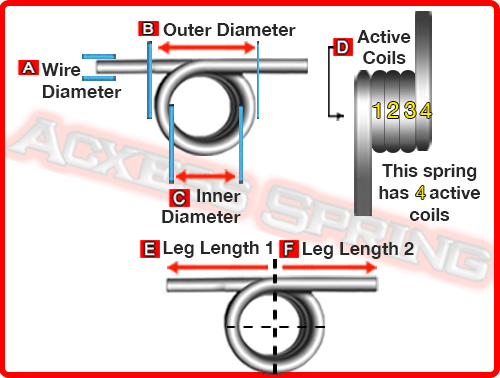For torsional springs, our torsion spring calculator requires that you input wire diameter, outer diameter, active coils, leg lengths, wind direction and material type. Like extension and compression springs, our torsional spring calculator will calculate all the other dimensions like torsion spring rate and free position when you hit the calculate button. Torsional springs usually go over a shaft so you have to be very conscious about your torsion spring’s inner diameter because it will contract (reduce) when your torsional spring is under torque.